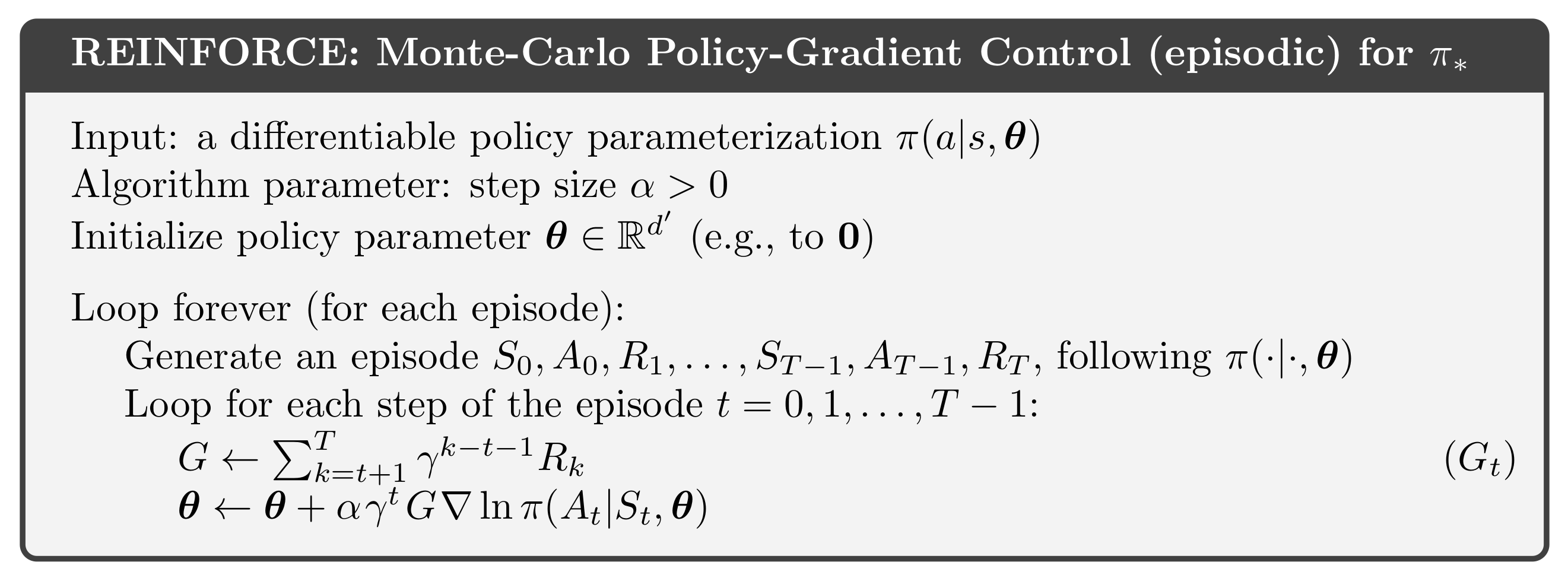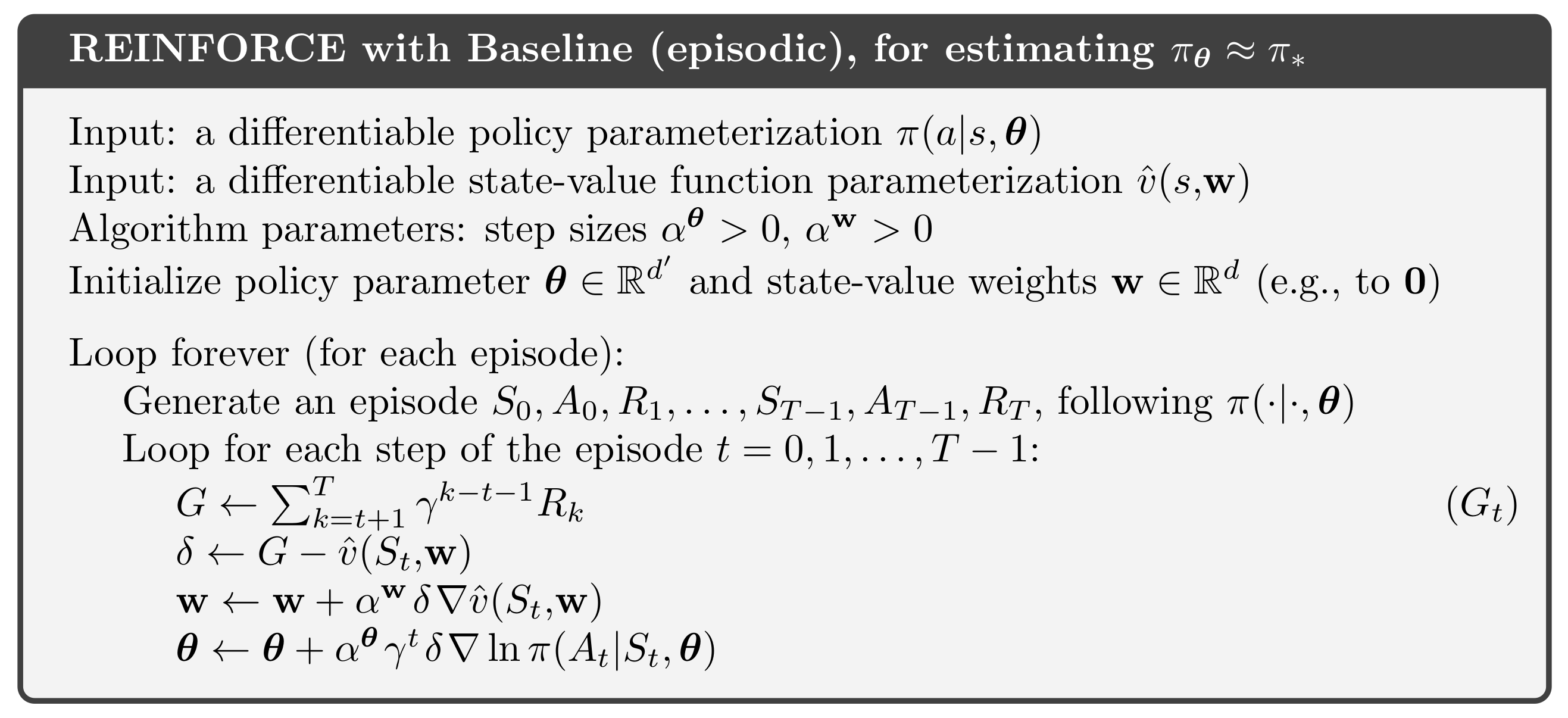# Unbiased Estimates in Policy Gradient

## Introduction

In reinforcement learning policy gradient methods, the goal is usually to maximize $\mathbb{E}[R_t]$, the expected value of return $R$ at time step $t$, where $R_t = \sum_{k=0}^{\infty} \gamma^k r_{t+k}$.

We have parameterized the policy $\pi(a|s; \theta)$ and update the parameter $\theta$ so that $\mathbb{E}[R_t]$ is maximized.

Standard REINFORCE algorithm updates policy in the direction $\nabla_{\theta} R_t \log \pi (a_t|s_t; \theta)$. That is to say, we have a loss function of $-\log R_t \pi (a_t|s_t; \theta)$ and we are minimizing the loss.But in order to mamixize $\mathbb{E}[R_t]$, why do we have to calculate the the derivatives for $R_t \log \pi (a_t|s_t; \theta)$? It turns out that it is because $\nabla_{\theta} R_t \log \pi (a_t|s_t; \theta)$ is an unbiased estimate of $\nabla_{\theta} \mathbb{E}[R_t]$, i.e., $\mathbb{E}[R_t \nabla_{\theta} \log \pi (a_t|s_t; \theta)] = \nabla_{\theta} \mathbb{E}[R_t]$.

## Proof

We use the definition of expected value, because random variable $R_t$ is dependent on $a_t$ and it follows distribution $\pi (a_t|s_t; \theta)$.

$$\nabla_{\theta} \mathbb{E}[R_t] = \nabla_{\theta} \int R_t \pi (a_t|s_t; \theta) d(a_t)$$

Then we apply a special case of Leibniz Integral Rule, where

$$\frac{d}{dx} \int_{a}^{b} f(x,t) dt = \int_{a}^{b} \frac{\partial}{\partial x} f(x,t) dt$$

We then have

$$\nabla_{\theta} \int R_t \pi (a_t|s_t; \theta) d(a_t) = \int \nabla_{\theta} [ R_t \pi (a_t|s_t; \theta) ] d(a_t)$$

Because $R_t$ is dependent on $a_t$ but not dependent on $\theta$ (Is it?). We have

$$\int \nabla_{\theta} [ R_t \pi (a_t|s_t; \theta) ] d(a_t) = \int R_t \nabla_{\theta} \pi (a_t|s_t; \theta) d(a_t)$$

We apply an identity trick here

\begin{aligned} \int R_t \nabla_{\theta} \pi (a_t|s_t; \theta) d(a_t) &= \int R_t \pi (a_t|s_t; \theta) \frac{\nabla_{\theta} \pi (a_t|s_t; \theta)}{\pi (a_t|s_t; \theta)} d(a_t) \\ &= \int R_t \pi (a_t|s_t; \theta) \nabla_{\theta} \log \pi (a_t|s_t; \theta) d(a_t) \end{aligned}

Because the random variable $R_t \nabla_{\theta} \log \pi (a_t|s_t; \theta)$ also follows distribution $\pi (a_t|s_t; \theta)$, we have

$$\int R_t \pi (a_t|s_t; \theta) \nabla_{\theta} \log \pi (a_t|s_t; \theta) d(a_t) = \mathbb{E}[R_t \nabla_{\theta} \log \pi (a_t|s_t; \theta)]$$

Therefore,

$$\nabla_{\theta} \mathbb{E}[R_t] = \mathbb{E}[R_t \nabla_{\theta} \log \pi (a_t|s_t; \theta)]$$

This concludes the proof.

## Generalization

More generally it is not hard to see that,

$$\nabla_{\theta} \mathbb{E}[f(z)] = \mathbb{E}[f(z) \nabla_{\theta} \log p (z; \theta)]$$

## Extension

We also use REINFORCE with baseline to reduce the variance of the estimate of $\nabla_{\theta} \mathbb{E}[R_t]$ while keeping the estimate unbiased.In stead of using $\nabla_{\theta} R_t \log \pi (a_t|s_t; \theta)$ as an unbiased estimate of $\nabla_{\theta} \mathbb{E}[R_t]$, we use $\nabla_{\theta} (R_t - v(s_t)) \log \pi (a_t|s_t; \theta)$ as long as $v(s_t)$ does not dependent on $a$.

To see why $\mathbb{E}[(R_t - v(s_t)) \nabla_{\theta} \log \pi (a_t|s_t; \theta)] = \nabla_{\theta} \mathbb{E}[R_t]$, we have

$$\nabla_{\theta} \mathbb{E}[R_t] = \nabla_{\theta} \int R_t \pi (a_t|s_t; \theta) d(a_t) = \nabla_{\theta} \int R_t \pi (a_t|s_t; \theta) d(a_t) - \nabla_{\theta} \int v(s_t) \pi (a_t|s_t; \theta) d(a_t)$$

Where $\nabla_{\theta} \int v(s_t) \pi (a_t|s_t; \theta) d(a_t) = 0$.

Because $v$ use different parameters other than $\theta$ and it is not dependent on $a$,

$$\nabla_{\theta} \int v(s_t) \pi (a_t|s_t; \theta) d(a_t) = v(s_t) \nabla_{\theta} \int \pi (a_t|s_t; \theta) d(a_t) = v(s_t) \nabla_{\theta} 1 = v(s_t) \times 0 = 0$$

## Quick Proof to Leibniz Integral Rule

\begin{aligned} \frac{d}{dx} \int_{a}^{b} f(x,t) dt &= \frac{d}{dx} \int_{a}^{b} \int_{\mathbf{x}} \frac{\partial f(x,t)}{\partial x} dx dt\\ &= \frac{d}{dx} \int_{\mathbf{x}} \int_{a}^{b} \frac{\partial f(x,t)}{\partial x} dt dx\\ &= \frac{d}{dx} \int_{\mathbf{x}} (\int_{a}^{b} \frac{\partial f(x,t)}{\partial x} dt) dx\\ &= \int_{a}^{b} \frac{\partial f(x,t)}{\partial x} dt\\ \end{aligned}

Thanks to the guidance from my friend Guotu Li on this.

## Final Remarks

There is a statement or an assumption we made during the proof, that is $R_t$ is not dependent on $\theta$. It is because in this case $R_t$ is a scala value instead of an output from the policy neural network.

Lei Mao

03-15-2019

03-15-2019